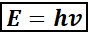# Problem: The human eye contains a molecule called 11-cis-retinal that changes shape when struck with light of sufficient energy. The change in shape triggers a series of events that results in an electrical signal being sent to the brain that results in vision. The minimum energy required to change the conformation of 11-cis-retinal within the eye is about 164 kJ/mol. Calculate the longest wavelength visible to the human eye.

###### FREE Expert Solution

Energy takes many forms including light, sound and heat. Different colors of light are given by photons of various wavelengths.

The physists Max Plank and Albert Einstein theorized that light was made of small packets of electromagnetic energy.

Each packet of energy is referred to as a photon.

• The energy of the radiation can be calculated using the equation,
•Where,

E is energy in J per photon

h is Planck’s constant (6.626 x 10 -34 J.s)

v is the frequency in Hz or s-1

92% (408 ratings)###### Problem Details

The human eye contains a molecule called 11-cis-retinal that changes shape when struck with light of sufficient energy. The change in shape triggers a series of events that results in an electrical signal being sent to the brain that results in vision. The minimum energy required to change the conformation of 11-cis-retinal within the eye is about 164 kJ/mol. Calculate the longest wavelength visible to the human eye.

Frequently Asked Questions

What scientific concept do you need to know in order to solve this problem?

Our tutors have indicated that to solve this problem you will need to apply the The Energy of Light concept. You can view video lessons to learn The Energy of Light. Or if you need more The Energy of Light practice, you can also practice The Energy of Light practice problems.

What is the difficulty of this problem?

Our tutors rated the difficulty ofThe human eye contains a molecule called 11-cis-retinal that...as medium difficulty.